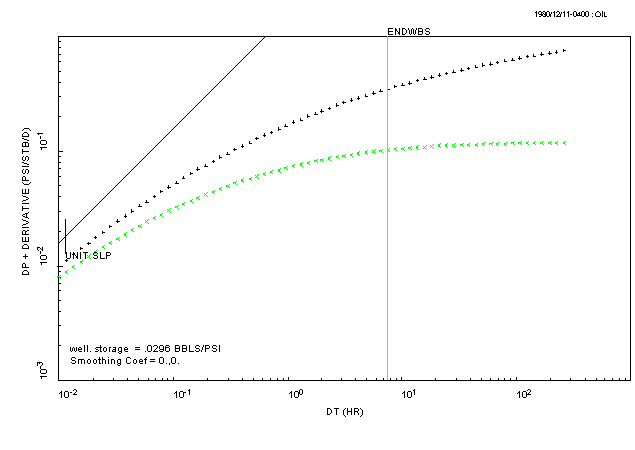## ESTIMATE THE WELL-BORE STORAGE COEFFICIENT

Select the ANALYSIS pull-down menu in PIE and select the "DIAGNOSTIC" option. This will display a set of graphics functions (the floating 'toolbar' at the bottom of the main window) that allow you to draw lines on the derivative plot.

When a derivative plot does not show a "hump", the odds are the well has been "stimulated" (e.g. a hydraulic-fracture treatment was done). In this situation, there is some "artistry" in estimating the well-bore storage coefficient which requires a bit of imagination on your part.

Select the button for the function called "UNITSL" and then move the mouse over the first data point on the UPPER curve. Now this is where you have to be artistic: pick a point a little above the data such that the curve of the data would extend back and follow the unit-slope line (I did say use your imagination...). The end result should look something like this:The meaning of the "ENDWBS" time marker gets a bit fuzzy for this type of derivative plot. Usually, this marker is the start of radial-flow in the reservoir (but then again, it may not). DO NOT interpret the "ENDWBS" marker as a 'hard' value dividing the data into good and bad data. The data prior to the ENDWBS marker should be included in the analysis!

Q: Is the storage-coefficient calculated from the unit-slope line reasonably close to C(min) and C(max)? A factor of 2 is "reasonably close".